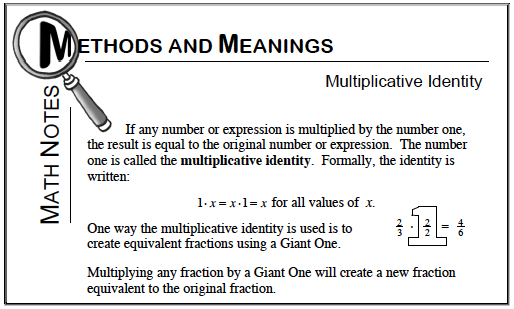### Home > MC1 > Chapter 7 > Lesson 7.1.1 > Problem7-10

7-10.

For each of the following problems, complete the fraction on the right so that the fractions are equal. Be sure to show your work clearly.

1.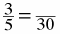2.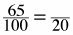3.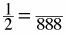Refer to the Math Notes box from Lesson 6.3.3 below.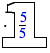$\quad \frac { 1 } { 2 } = \frac { 1 } { 888 }$

Refer to parts (a) and (b).